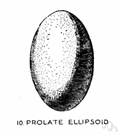# ellipsoid

(redirected from Franz Schweigger-Seidel)
Also found in: Thesaurus, Medical, Encyclopedia.

## el·lip·soid

(ĭ-lĭp′soid′)
n.
A geometric surface, all of whose plane sections are either ellipses or circles.

el·lip′soid′, el·lip′soi′dal (ĭ-lĭp′soid′l, ĕl′ĭp-, ē′lĭp-) adj.

## ellipsoid

(ɪˈlɪpsɔɪd)
n
(Mathematics)
a. a geometric surface, symmetrical about the three coordinate axes, whose plane sections are ellipses or circles. Standard equation: x2/a2 + y2/b2 + z2/c2 = 1, where ±a, ±b, ±c are the intercepts on the x-, y-, and z- axes
b. a solid having this shape: the earth is an ellipsoid.

## el•lip•soid

(ɪˈlɪp sɔɪd)

n.
1. a solid figure whose plane sections are all ellipses or circles.
 Noun 1ellipsoid - a surface whose plane sections are all ellipses or circles; "the Earth is an ellipsoid"plane figure, two-dimensional figure - a two-dimensional shape Adj. 1 ellipsoid - having the nature or shape of an ellipsoidrounded - curving and somewhat round in shape rather than jagged; "low rounded hills"; "rounded shoulders"